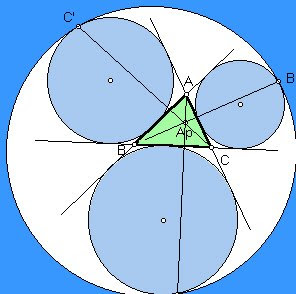## Sunday, 19 October 2008

### Even More on Pythagorean Triples and Barning TreesA while back I wrote a couple of blogs on Primitive Pythagorean triples and Matrices and Barning Trees of Primitive Pythagorean triples. Shortly afterward I got a nice note from H. Lee Price with a link to a paper he wrote on "The Pythagorean Tree: A New Species". A couple of things I learned from it were too good to keep to myself, so here are some of the things I thought were amazing.. or read the paper for yourself to find your own favorites.
One of the clever things that I learned was a way of creating an informative 2x2 matrix for any triple that holds some interesting information. I will use the 5,12,13 triangle as an example, but any of them produce the same sorts of information. Take one leg, and write it as a ratio to the sum of the hypotenuse and other leg, then simplify. Using 5 over 12+13 gives 5/25 or 1/5. Using the other leg we get 12/18 = 2/3. One of the first amazing things is that there will always be one, and only one even number in the two fractions created. Put the numerator and denominator of the fraction with the even number in the left column of a 2x2 matrix, and the other fraction makes the right column. For the 5, 12, 13 Pythagorean triple we get:"SO WHAT?", you ask. Well, amazingly, you can use these to quickly find the radii of the in-circle, and all three ex-circles of the triangle. If you multiply across the two rows the two products formed will give you the radius of the in-circle and also the radius of the ex-circle on the hypotenuse of the triangle. Then if you multiply the two diagonals, you get the other two ex-circles radii. In the case of the 5,12, 13 triangle, the in-circle has a radius of 2, and the three ex-circles have radii of 3, 10, and 15 units respectively. I had never observed until it was pointed out to me, that the radius of the ex-circle on the hypotenuse is always the sum of the radii of the in-circle and the other two ex-circles...2 + 3 + 10 = 15....
Finally, these in-circle and ex-circle relations are tied back to the Barning tree. Remember the diagram that shows that each of the Pythagorean triples produces three offspring in the tree. Mr. Price points out that in each case, the new Triangle has an in-circle that is one of the three ex-circles of the parent triangle. The 3,4,5 triangle has ex-circle radii of 2, 3 and 6 units. The off spring triangles are the 5, 12, 13 triangle with in-center radius of 2, the 8, 15, 17 triangle with in-center radius of 3, and the 20,21,29 triangle with in-center radius of 6.
Somehow, all that geometry packed into a simple matrix seems incredible. By the way, the original two fractions we used to form the matrix, they are the tangents of 1/2 the two acute angles of the triangle. The focus of Mr. Price's paper was to point out that from these 2x2 matrices, you can easily build a different tree to produce all the Primitive Pythagorean triples as well. I will return to that when time permits, and I feel I understand it well enough to do it justice.

#### 1 comment:Anonymous said...

help me.